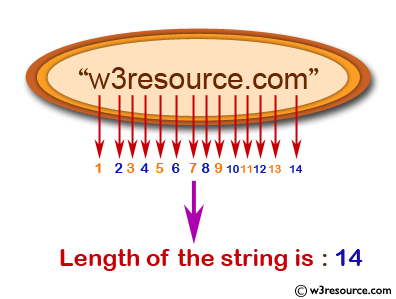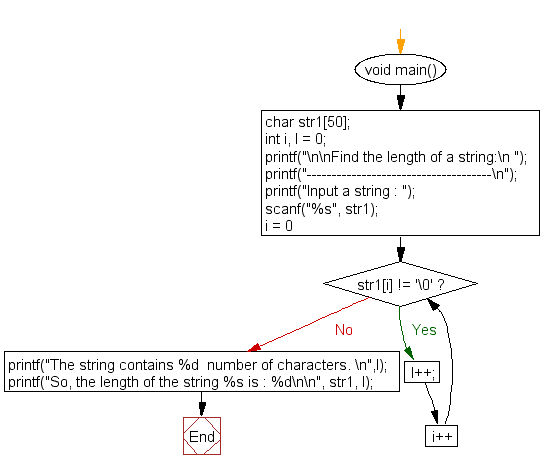﻿ C : Length of a string without using the library function# C Exercises: Find the length of a string without using the library function

## C For Loop: Exercise-58 with Solution

Write a C program to find the length of a string without using the library function.

Pictorial Presentation:Sample Solution:

C Code:

``````#include <stdio.h>
#include <string.h>

void main()
{
char str1;
int i, l = 0;

printf("\n\nFind the length of a string:\n ");
printf("-------------------------------------\n");

printf("Input a string : ");
scanf("%s", str1);

for (i = 0; str1[i] != '\0'; i++)
{
l++;
}
printf("The string contains %d  number of characters. \n",l);
printf("So, the length of the string %s is : %d\n\n", str1, l);
}
```
```

Sample Output:

```Find the length of a string:
-------------------------------------
Input a string : welcome
The string contains 7  number of characters.
So, the length of the string welcome is : 7
```

Flowchart:C Programming Code Editor:

Improve this sample solution and post your code through Disqus.

What is the difficulty level of this exercise?

Test your Programming skills with w3resource's quiz.

﻿